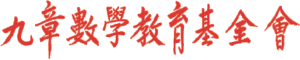首頁新聞區討論區檔案下載重要公告

 歷史公告

### 中學數學競賽

#### HOMC 2019

 主選單
 · 回首頁· 新聞區 · 討論區 · 檔案下載 · 網站連結 · 電子相薄 · 夥伴網站 · 精華文章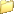討論區主頁10學習討論區請問離散學數的問題二則????? 限會員
 全部展開樹狀顯示 舊的在前 新的在前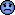請問離散學數的問題二則?????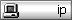第一題:Let A ={1,2,3,4}and B={a,b,c,d,e}.Let f:A→B be defined by f={(1,d),(2,e),(3,d),(4,b)} and g:B→A {(a,2),(b,1),(c,3),(d,1),(e,4)}.List the ordered paris in f。g and g。f .第二題:Let R be the relation on Z*N defined by (a,b) R (c,d) if ad=bc,then prove that R is an equivalence relation.麻煩各位高手了.......謝謝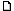2002-10-20 18:33Re: 請問離散學數的問題二則?????In First Problem:The function f。g :B -->B and g。f : A -->Athus, f。g={(a,e), (b,d), (c,d), (d,d), (e,b)}Try g。f yourself!The Second Proiblem:Let R be the relation on Z*N defined by (a,b) R (c,d) if ad=bc,then prove that R is an equivalence relation.Proof:1. since aa=aa, => (a,a) R (a,a) for (a,a) in Z*N2. If (a,b) R (c,d) then ad=bc => bc=ad, thus (a,b) R (c,d).3. If (a,b) R (c,d), and (c,d) R (e,f), for all of them are in Z*N, then we have ad=bc and de=cf,since af=ade/c = bce/c = be,thus (a,b) R (e,f).by 1.~3. we have prove that the Relation R is an equi. relation on Z*N.2002-10-22 09:42
 全部展開樹狀顯示 舊的在前 新的在前

 選擇討論區   九章數學基金會 ---------------- 讀書討論區 2012國際數學競賽專區   數學討論區 ---------------- 學習討論區 數學玩具討論區 小學 國中 高中 教學 其他 家長   九章數學教育基金會各項活動的感想與建議 ---------------- 參加小學數學競賽的感想與建議 參加中學數學競賽的感想與建議 參加九章數學俱樂部的感想與建議 參加奧林匹克訓培營的感想與建議 參加加拿大英文及數學夏令營的感想與建議 其它九章數學教育基金會活動的感想與建議   站內事務區 ---------------- 本站使用協助與建議區   九章數學愛好者聯誼會員專區 ---------------- 九章數學愛好者聯誼會員專區九章數學出版社、九章數學基金會版權所有本網頁各鍊結標題及鍊結內容歸原權利人所有Copyright 2000 ~2004九章數學出版社、九章數學基金會本網站內所有文字及資料版權均屬九章所有，未經書面同意之商業用途必究This web site was made with XOOPS, a web portal system written in PHP. XOOPS is a free software released under the GNU/GPL license.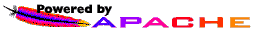Powered by XOOPS 1.3.10 © 2002 The XOOPS Project